## Squarefree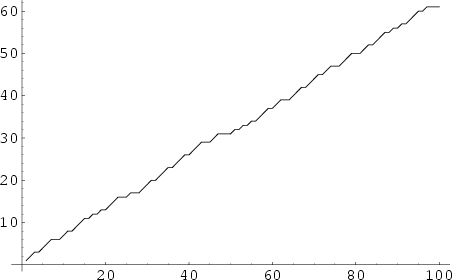A number is said to be squarefree (or sometimes Quadratfrei; Shanks 1993) if its Prime decomposition contains no repeated factors. All Primes are therefore trivially squarefree. The squarefree numbers are 1, 2, 3, 5, 6, 7, 10, 11, 13, 14, 15, ... (Sloane's A005117). The Squareful numbers (i.e., those that contain at least one square) are 4, 8, 9, 12, 16, 18, 20, 24, 25, ... (Sloane's A013929).

The asymptotic number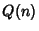of squarefree numbers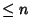is given by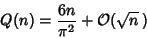(1)

(Hardy and Wright 1979, pp. 269-270).for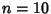, 100, 1000, ... are 7, 61, 608, 6083, 60794, 607926, ..., while the asymptotic density is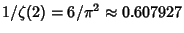, whereis the Riemann Zeta Function.

The Möbius Function is given by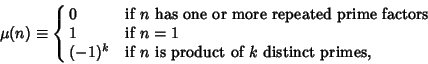(2)

so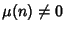indicates thatis squarefree. The asymptotic formula for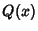is equivalent to the formula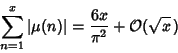(3)

(Hardy and Wright 1979, p. 270)

There is no known polynomial-time algorithm for recognizing squarefree Integers or for computing the squarefree part of an Integer. In fact, this problem may be no easier than the general problem of integer factorization (obviously, if an integercan be factored completely,is squarefree Iff it contains no duplicated factors). This problem is an important unsolved problem in Number Theory because computing the Ring of integers of an algebraic number field is reducible to computing the squarefree part of an Integer (Lenstra 1992, Pohst and Zassenhaus 1997). The Mathematica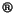(Wolfram Research, Champaign, IL) function NumberTheoryNumberTheoryFunctionsSquareFreeQ[n] determines whether a number is squarefree.

All numbers less than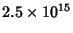in Sylvester's Sequence are squarefree, and no Squareful numbers in this sequence are known (Vardi 1991). Every Carmichael Number is squarefree. The Binomial Coefficients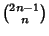are squarefree only for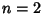, 3, 4, 6, 9, 10, 12, 36, ..., with no others less than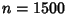. The Central Binomial Coefficients are Squarefree only for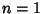, 2, 3, 4, 5, 7, 8, 11, 17, 19, 23, 71, ... (Sloane's A046098), with no others less than 1500.

See also Binomial Coefficient, Biquadratefree, Composite Number, Cubefree, Erdös Squarefree Conjecture, Fibonacci Number, Korselt's Criterion, Möbius Function, Prime Number, Riemann Zeta Function, Sárközy's Theorem, Square Number, Squareful, Sylvester's Sequence

References

Bellman, R. and Shapiro, H. N. The Distribution of Squarefree Integers in Small Intervals.'' Duke Math. J. 21, 629-637, 1954.

Hardy, G. H. and Wright, E. M. The Number of Squarefree Numbers.'' §18.6 in An Introduction to the Theory of Numbers, 5th ed. Oxford, England: Clarendon Press, pp. 269-270, 1979.

Lenstra, H. W. Jr. Algorithms in Algebraic Number Theory.'' Bull. Amer. Math. Soc. 26, 211-244, 1992.

Pohst, M. and Zassenhaus, H. Algorithmic Algebraic Number Theory. Cambridge, England: Cambridge University Press, p. 429, 1997.

Shanks, D. Solved and Unsolved Problems in Number Theory, 4th ed. New York: Chelsea, p. 114, 1993.

Sloane, N. J. A. A013929, A046098, and A005117/M0617 in An On-Line Version of the Encyclopedia of Integer Sequences.'' http://www.research.att.com/~njas/sequences/eisonline.html.

Vardi, I. Are All Euclid Numbers Squarefree?'' §5.1 in Computational Recreations in Mathematica. Reading, MA: Addison-Wesley, pp. 7-8, 82-85, and 223-224, 1991.